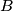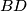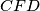### IMO Shortlist 1994 problem G1

Kvaliteta:
Avg: 0,0
Težina:
Avg: 6,0$C$ and$D$ are points on a semicircle. The tangent at$C$ meets the extended diameter of the semicircle at$B$, and the tangent at$D$ meets it at$A$, so that$A$ and$B$ are on opposite sides of the center. The lines$AC$ and$BD$ meet at$E$.$F$ is the foot of the perpendicular from$E$ to$AB$. Show that$EF$ bisects angle$CFD$
Izvor: Međunarodna matematička olimpijada, shortlist 1994